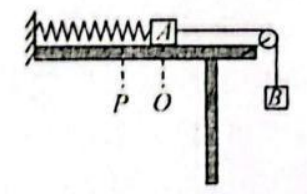$\text{A.}$ 由 $P$ 点释放物体 $A$ 瞬间,物体 $A$ 的加速度为零 $\text{B.}$ 绳子能承受的最大拉力为 $\frac{10}{3} m g$ $\text{C.}$ 从 $P$ 点到 $O$ 点,物体 $A$ 劫能的增加量小于重力对物体 $B$ 所做功与弹簧弹力对 $A$ 做的 功之和 $\text{D.}$ 从 $P$ 点到 $O$ 点,物体 $A$ 与弹簧所组成的系统机械能的增加量等于物体 $B$ 所受重力对 $B$ 做的功

BC